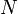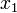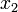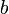# Exercise: Congestion Control Stability Analysis

A simple model for congestion control betweencomputers connected by a router is given by the differential equation==where,are the transmission rates for the sources of data,is the current buffer size of the router,is the maximum buffer size, andis the capacity of the link connecting the router to the computers. Theequation represents the control law that the individual computers use to determine how fast to send data across the network (this version is motivated by a protocol called "Reno") and theequation represents the rate at which the buffer on the router fills up.
Consider the case where(so that we have three states,,, and), and takeMb andMb/sec. Locate the equilibrium points for the system and indicate whether each is asymptotically stable, stable (but not asymptotically stable), or unstable.